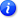Restore Prior EntriesAML Composite Model Calculator

Instructions
• Each section has additional instructions which you can display by clicking on the section'sicon.
• Please check a box or enter a value as it applies to the patient's condition- keeping in mind that in order the for the model to be accurate, all necessary components need to be entered.
• Click on one of the buttons at the bottom of the page to calculate the score or save your entries for later.
You need to correct the following errors before a score can be calculated:
A) Augmented HCT-CI
1. Arrhythmia (score = 1)a.
Yes
b.
Yes
c.
Yes
d.
Yes
e.
Yes
f.
Yes
g.

Yes
2. Cardiovascular Score (score = 1)a.
Yes
b.
Yes
c.
%
d.
%
3. Inflammatory Bowel Disease (score = 1)a.
Yes
b.
Yes
4. Diabetes (score = 1)a.
Yes
b.
Yes
5. Cerebro-Vascular Disease (score = 1)a.
Yes
b.
Yes
c.
Yes
d.
Yes
e.
Yes
6. Psychiatric Disturbance (score = 1)a.
Yes
b.
Yes
c.

Yes
7. Hepatic Comorbidity (score = 1 or 3)a.
Total bilirubin:
b.
Aspartate transaminase (AST):
c.
Alanine transaminase (ALT):
d.
Alkaline Phosphatase (ALK):
e.
Yes
f.
Yes
g.
Yes
8. Obesity (score = 1)a.
Calculated BMI:
b.
%
9. Infection (score = 1)a.
Yes
b.
Yes
c.
Yes
d.
Yes
e.

Yes
10. Rheumatologic Score (score = 2)a.
Yes
b.
Yes
c.
Yes
d.
Yes
e.
Yes
f.

Yes
11. Peptic Ulcer (score = 2)a.
Yes
b.
Yes
12. Renal Comorbidity (score = 2)a.
ml/min
b.
Serum Creatinine
Please select units and enter value
i.
ii.
c.
Yes
d.
Yes
13. Pulmonary Score (score = 2 or 3)a.
Diffusion capacity of carbon monoxide (DLco)
Please enter items i and ii
i.
%
ii.
g/dl
iii.
b.
Forced expiratory volume in 1 second (FEV1)
i.
%
c.
Yes
d.
Yes
e.
Yes
14. Prior Solid Tumor (score = 3)a.

Yes
15. Heart Valve Disease (score = 3)a.

Yes
b.

Yes
c.

Yes
d.

Yes
16. Hypoalbuminemia (score = 1)a.
17. Elevated LDH (score = 1 or 2)a.
U/L

B) Patient Age
Patient Age

C) Cytogenetic-molecular risks per ELN 2017 Classification
A.
I.
Yes
II.
Yes
III.
Yes
IV.
i.
No or
ii.
Yes
V.
Yes
B.
I.
i.
Yes or
ii.
Yes
II.
i.
No
ii.
Yes
III.
Yes
IV.
Yes
C.
I.
Yes
II.
Yes
III.
Yes
IV.
Yes
V.
Yes
VI.Yes
VII.Yes
VIII.
Yes
IX.
Yes
X.
Yes
XI.
Yes